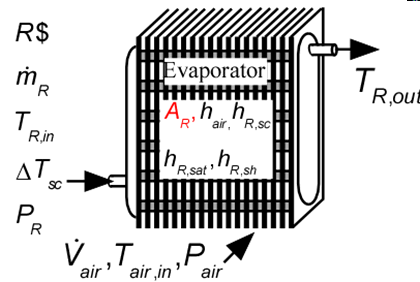Contents - index

Evaporator1_CLEvaporator1_CL determines the required heat transfer surface area to evaporate a two-phase refrigerant to a specified degree of superheat by heat exchanger with air.  No consideration is provided for condensation or frost formation in this routine.  It is necessary to provide the heat transfer coefficients on the air side of the heat exchanger and for the saturated, and superheated sections on the refrigerant side.  These heat transfer coefficients can be determined using the functions in the Heat Transfer Library, as illustrated in the example.

This library file can be used in the design stage of the evaporator by providing the design inlet conditions to determine the required evaporator area.  Use Evaporator2_CL to determine the refrigerant and air outlet states for given inlet conditions and evaporator area.

Inputs:

R\$:   name of the refrigerant

m_dot_R:  refrigerant flow rate (kg/s, lbm/hr)

h_R_in:  specific enthalpy of the entering refrigerant (J/kg, kJ/kg, Btu/lbm)

P_R: pressure of the entering refrigerant

DELTAT_sh:  number of degrees of superheat (C, K, F, R)

V_dot_air:  volumetric flow rate of air (m^3/s, cfm)

T_air_in:  air inlet temperature  (C, K, F, R)

P_air:  air pressure (Pa, kPa, bar, MPa, psia, atm)

h_air:  effective outside (air-side) heat transfer coefficient per unit refrigerant tube area (W/m^2-K, Btu/hr-ft^2-R)

A_air\A_R:  ratio of effective heat transfer area on the air side to the heat transfer area for the refrigerant*

h_R_sat:  average heat transfer coefficient for refrigerant in the saturated regime  (W/m^2-K, Btu/hr-ft^2-R)

h_R_SH:  heat transfer coefficient for refrigerant in the superheat regime  (W/m^2-K, Btu/hr-ft^2-R)

Outputs:

A_R:  required heat transfer area for the refrigerant (m^2, ft^2)

UA:  overall heat transfer coefficient (W/K ,Btu/hr-R)

Q_dot:  overall heat transfer rate (W, kW, Btu/hr)

h_R_out outlet specific enthalpy of the refrigerant  (J/kg, kJ/kg, Btu/lbm)

T_air_out:  outlet temperature of the air (C, K, F, R)

f_sh:  fraction of the evaporator area that is in the superheat section

*A_air\A_R is the ratio of the product of the overall fin efficiency and total (finned and unfinned) area on the air side to the total surface area inside the refrigerant flow passages.  For unfinned surfaces, A_air\A_R is the ratio of the outer surface to inner surface areas.

Example:

\$UnitSystem SI C kPa mass kJ

\$TabStops 0.2 4 in

R\$='R134a' "refrigerant"

m_dot_R=0.008 [kg/s]

T_cond_out=38 [C] "condenser exit temperature"

P_cond=1 [MPa]*convert(MPa,kPa) "condenser pressure"

h_cond_out=enthalpy(R\$,T=T_cond_out,P=P_cond) "specific enthalpy of refrigerant exiting condenser"

P_R=175 [kPa] "evaporator pressure"

T_R_sat=T_sat(R\$,P=P_R) "evaporator saturation temperature"

h_R_in=h_cond_out "specific enthalpy of refrigerant entering evaporator"

x_R_in=quality(R\$,h=h_R_in,P=P_R) "inlet quality"

T_air_in=5 [C] "air inlet temperature"

P_air=101.3 [kPa] "air pressure"

V_dot_air=0.08 [m^3/s] "volumetric flow rate of air"

DELTAT_sh=5 [DELTAK] "degrees of superheat"

h_R_sh=315 [W/m^2-K] "convection coefficient for superheated R134a.  See calculation of h_T_sh below"

h_R_sat=1000 [W/m^2-K] "convection coefficient for saturated R134a - see calculation of h_cond below"

h_air=53.2 [W/m^2-K] "convection coefficient for air.  See calculation of h_air1 below"

A_air\A_R=A_air\A_R1 "ratio of area on the air side to that on the refrigerant side"

Call Evaporator1_CL(R\$, m_dot_R, h_R_in, P_R, DELTAT_sh, V_dot_air, T_air_in, P_air, h_air, A_air\A_R,  h_R_sat, h_R_sh : A_R, UA, Q_dot, h_R_out, T_air_out, f_sh)

"!Calculation of the heat transfer coefficients using the Heat Transfer Library functions"

\$VarInfo A_air\A_R Guess=10  Lower=1 Upper=100

N_circuits=1 "number of parallel circuits"

A_fr=0.26*0.2 [m^2] "frontal area"

W=0.2 [m] "length of tubes"

N_tubes=A_R/(pi*W*D_in) "required number of tubes"

Call CHX_geom_finned_tube('fc_tubes_s80-38T': D_o, fin_pitch, D_h, fin_thk, sigma, alpha, A_fin\A) "air side geometry"

"Determine ratio of area on air side to the refrigerant heat transfer area"

th = 0.9 [mm]*convert(mm,m) "tube wall thickness"

D_in=D_o-2*th "inner tube diameter"

A_unfinned=pi*D_o*N_tubes*W*(1-fin_thk*fin_pitch) "unfinned area on air side"

A_tot=A_unfinned+A_finned "total finned and unfinned area on air side"

A_finned/A_tot=A_fin\A "ratio of finned to total area is provided by Compact HX library"

eta_o=0.97 "overall fin efficiency - See Heat Transfer, Nellis and Klein, section 8.5"

A_air\A_R1=eta_o*A_tot/(N_tubes*W*pi*D_in) "ratio of heat transfer area on air side to that on refrigerant side"

"Determine heat transfer coefficients for air and refrigerant "

m_dot_air=V_dot_air/volume(air,T=T_air_in,P=P_air) "air mass flow rate"

Call CHX_h_finned_tube('fc_tubes_s80-38T', m_dot_air, A_fr, 'Air', T_air_in, P_air: h_air1) "compact hx correlation"

call PipeFlow('R134a',T_R_sat+1 [C], P_R, m_dot_R/N_circuits, D_in, N_tubes*W*f_sh, 0: h_T_sh, h_H_sh, DELTAP_sh, Nusselt_T_sh, f_R_sh, Re_R_sh) "superheat section"

T_w=T_air_in "estimated wall temperature"

G=m_dot_R/N_circuits/(pi*D_in^2/4) "mass velocity"

q``=UA/A_R*(T_air_in-T_R_sat) "heat flux"

h_cond=Flow_Boiling_avg(R\$, T_R_sat, G, D_in, x_R_in, 1, q``, 'Horizontal') "saturated section"

{Solution:

A_R=0.2495 [m^2]

f_sh=0.05304

Q_dot=1.131 [kW]

T_air_out=-6.101 [C]

T_R_out=-8.423 [C]

UA=95.49 [W/K]

}